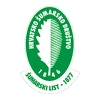DIGITALNA ARHIVA ŠUMARSKOG LISTA
prilagođeno pretraživanje po punom tekstu

 ŠUMARSKI LIST 7-8/2023 str. 59     <-- 59 -->        PDF selecting satellite images is for the image to contain little or no clouds and haze, and belong to different seasons in order to discern the phenological phases of plants (Abdi 2020). The matching of the spatial resolution of 20 m with the spatial resolution of 10 m is performed based on the method of the nearest neighbor element in the R programming language. Calculating NDVI, raster classification, accuracy assessment of classifications, and computational analysis of raster differences were performed using the R programming language, whereas QGIS was used for creating training sets, validation sets, and visual analysis. NDVI was calculated in R based on the quotient of the difference between the red and infrared bands and the sum of those bands (Rouse Jr et al. 1973):                 NDVI=(NIR-RED)/(NIR+RED),               1 This value represents the normalized difference of vegetation indices and is determined based on spectral values from channels B8 (Near Infrared) and B4 (Red). NDVI values were calculated for both series of images, and one raster representing the difference between the two NDVI images was also calculated. For the purpose of training, data was prepared from the area sourced from the Copernicus platform. Data preparation was performed in the QGIS software environment. Training data are crucial components in supervised learning and most machine learning algorithms require a large number of training data samples (Abdi 2020). A higher number of carefully selected samples bring better final results. In the area where it is necessary to perform the classification based on the calculated values of NDVI, polygons were drawn according to the previously established classes (forest, other). For each class, about 20 polygons were drawn on different parts of the raster. It was important to consider the size of the drawn polygon to ensure that the given polygons are the best possible representation of the given class. After the preparation of the training set, RF was applied to classify images. The model process has been carried out using loaded training data, predictors, and the desired learning technique, as well as defining metric criteria and determining the best model. The Kappa coefficient was used as a criterion for determining the best model. After cross-validation, it was possible to view the results with the best-obtained parameters through the parameter optimization process. After the classification was completed, it was important to calculate the accuracy of the results. Accuracy was defined as the degree to which the map produced agrees with the reference classification (Olofsson et al. 2013). Independent data collection was important to determine the accuracy and credibility of spatial information. Therefore, there were two sets of data; the first set was used to create a classification model (training set), which was then applied to the second set (validation set). Performance measures were determined by comparing the classified and reference (known) output classes on the validation data set, which could be represented by a confusion matrix (Kovačević 2022). Based on confusion matrix, it was possible to calculate various quantitative measures: OA (Overall Accuracy), PA (Producer’s Accuracy), UA (User’s Accuracy), and Kappa coefficient of agreement (Liu et al. 2007). The Kappa coefficient can have a value from -1 to 1. A kappa coefficient ranging from 0.81 to 1.00 indicates a very good agreement (Miranda et al. 2018). Data validation was performed in the QGIS software package. Polygons were drawn on the given area independently of the classified raster and training data. The points were intersected with the newly created polygons, and the values for the classified area were obtained. After loading the appropriate data, the confusion matrix and other parameters for accuracy assessment were calculated. The accuracy achieved by raster classification for 2017 and 2022 is shown in Table 1. Data from the Real Estate Cadastre Database of Brezici were obtained on October 25, 2022. As input data, .shp files were used, which were manipulated in the QGIS software package. The classification of vector data was performed into two classes: forest, and other. After creating the classes, the vector data were converted into a raster.